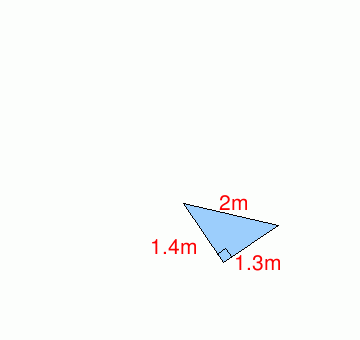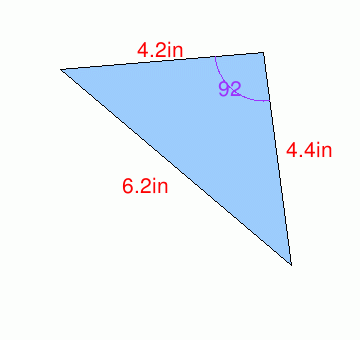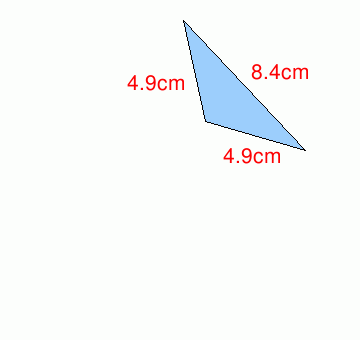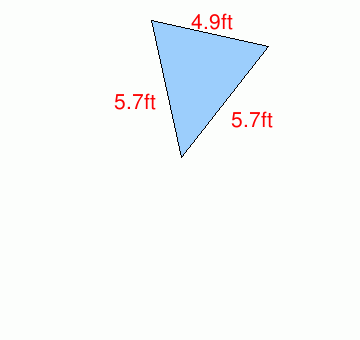MathScore EduFighter is one of the best math games on the Internet today. You can start playing for free!

## Triangle Types - Sample Math Practice Problems

The math problems below can be generated by MathScore.com, a math practice program for schools and individual families. References to complexity and mode refer to the overall difficulty of the problems as they appear in the main program. In the main program, all problems are automatically graded and the difficulty adapts dynamically based on performance. Answers to these sample questions appear at the bottom of the page. This page does not grade your responses.

See some of our other supported math practice problems.

### Complexity=1

Determine whether the triangle is equilateral, isosceles, or scalene based on the length of the sides, and whether it is an acute, right, or obtuse triangle based on the angles.

 1Type (based on sides): --- equilateral isosceles scalene Type (based on angles): --- acute right obtuse 2Type (based on sides): --- equilateral isosceles scalene Type (based on angles): --- acute right obtuse

### Complexity=2

Determine whether the triangle is equilateral, isosceles, or scalene based on the length of the sides, and whether it is an acute, right, or obtuse triangle based on the angles.

 1Type (based on sides): --- equilateral isosceles scalene Type (based on angles): --- acute right obtuse 2Type (based on sides): --- equilateral isosceles scalene Type (based on angles): --- acute right obtuse

### Complexity=1

Determine whether the triangle is equilateral, isosceles, or scalene based on the length of the sides, and whether it is an acute, right, or obtuse triangle based on the angles.

1Type (based on sides):
Type (based on angles):
2Type (based on sides):
Type (based on angles):

### Complexity=2

Determine whether the triangle is equilateral, isosceles, or scalene based on the length of the sides, and whether it is an acute, right, or obtuse triangle based on the angles.

12